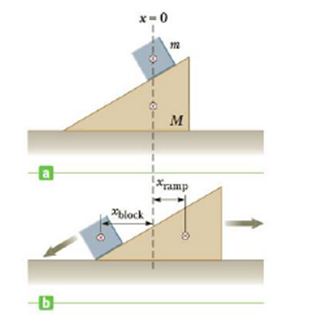Chapter 8, Problem 13P

Chapter
Section
Textbook Problem

A block of mass m = 1.50 kg is at rest on a ramp of mass M = 4.50 kg which, in turn, is at rest on a friction less horizontal surface (Fig. P8.13a). The block and the ramp are aligned so that each has its center of mass located at x = 0. When released, the block slides down the ramp to the left and the ramp, also free to slide on the frictionless surface, slides to the right as in Figure P8.13b. Calculate xramp, the distance the ramp has moved to the right, when xblock = −0.300 m.Figure P8.13

To determine
The distance the ramp has moved to the right.

Explanation
Assume that the center of mass of the block-ramp system is unchanged and there is no external force on the system along the horizontal direction. For the distance , the ramp moved due to the motion of the block, the concept of center of mass is used to calculate it.

Given info: The x coordinate of the center of gravity of the block-ramp system is 0m , the distance the block moved left on the ramp is 0.300m , the mass of the block is 1.50kg , and the mass of the ramp is 4.50kg .

The formula for the distance that the ramp has moved to the right is,

xcm=mxb+Mxrm+M

• m is mass of the block.
• M is mass of the ramp.
• xb is the distance moved by the block to the left.
• xr is the distance moved by the ramp to the right.

Substitute 4.50kg for M , 1.50kg for m , 0

Still sussing out bartleby?

Check out a sample textbook solution.

See a sample solution

The Solution to Your Study Problems

Bartleby provides explanations to thousands of textbook problems written by our experts, many with advanced degrees!

Get Started

Find more solutions based on key concepts